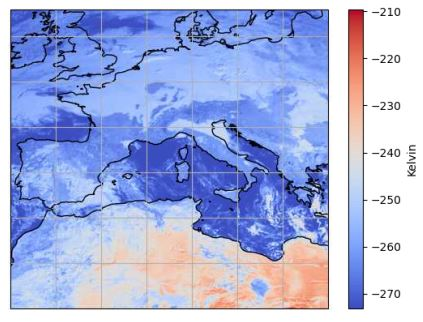# Get pixel rgb value

My name is Ger from the Netherlands and have a question:
I have this : area = new_scn[chan].attrs[‘area’]
How I can I get the pixel RGB value from scene new_scn[chan] by coordinate or row,col from image

Thanks for moving this here. Can you give us more complete code with imports, including what new_scn is? That doesn’t particularly look like matplotlib.

Again sorry and glad that this is possible. I have data from weather satellite and via Satpy process the decoding of the scene to image. I also process this data with Matplotlib to create a temperature map. In this map I can add places based on coordinators, but I also want to process the temperature in an excel sheet. That’s why I need the pixel value. I also want to read the plot generated by Matplotlib the pixel color for comparison or possibly adjust.

new_scn[chan] is the scene of the channel processed by Satpy and this is where Matplotlib takes over.

Nu de script
crs = new_scn[chan].attrs[‘area’].to_cartopy_crs()
ax = plt.axes(projection=crs)

ax.coastlines()
ax.gridlines()
ax.set_global()
chan_data = new_scn[chan]-273.15 # plot data
im = plt.imshow(chan_data, transform=crs, extent=crs.bounds, origin=‘upper’, cmap=“coolwarm”)
cbar = plt.colorbar()
cbar.set_label(“Kelvin”)

lon = 4.666
lat = 52.496
area = new_scn[chan].attrs[‘area’]
print('lon-lat ',lon, lat)
col, row = area.get_xy_from_lonlat(lon, lat)
print('col-row ',col, row)

global temp
temp = int(new_scn[chan].values[row, col]-273.15) #<-- Here i read the data of the pixel
print('temp-col-row ',temp, col, row)

HERE I WANT TO READ THE RGB VALUEOF THE PIXEL

plt.show()

I hope I come across clearly and you can help me

Ger

You want to know what color of the `coolwarm` colormap your data maps to?

``````norm = im.norm
cmap=im.cmap
rgb= cmap(norm(data))
``````

should work, where data is one of your pixels.

Thanks for the fast replay and help.
I get color numbers, perfect
One small question, can you tell me how to translate the numbers
rgb = [[0.70567316 0.01555616 0.15023281 1. ]
[0.70567316 0.01555616 0.15023281 1. ]]

dear jklymak ,
I think i do something wrong because every time i get the same value.

norm = im.norm
cmap=im.cmap
data = row,col
rgb= cmap(norm(data))
print('rgb = ', rgb)

What do i wrong?

Impossible for us to know if you don’t tel us how you are specifying `data`

I have coordinate lon = 4.666 lat = 52.496
I made an area → area = new_scn[chan].attrs[‘area’]
I made column and row from the coordinates → col, row = area.get_xy_from_lonlat(lon, lat)
And that coordinate i wil read from the plot.

Same as i read the temperature value from the pixel (temp = int(new_scn[chan].values[row, col]-273.15) ), i ask if it is possible to read also the RGB color value.

I will just offer generic advice here - use lots of `print` statements and try to figure out what is going on.

@jklymak , one other question.
I install complete new Satpy installation on Raspberry Pi. It works but one thing:
When the script have to make image from Satpy scene the program stops with no error.
I write in Python:
image = np.asarray(local_scn[“natural_color”]).transpose(1,2,0)
After this the program stops with:
=============================== RESTART: Shell ===============================
What do i have to install to make ik work oke.

The old installation have no problems.